# Time SummaryHi,

I need to have a SUM summary for a line item but it doesn't add up but results to zero instead.

I attached a screenshot for reference. Thank you.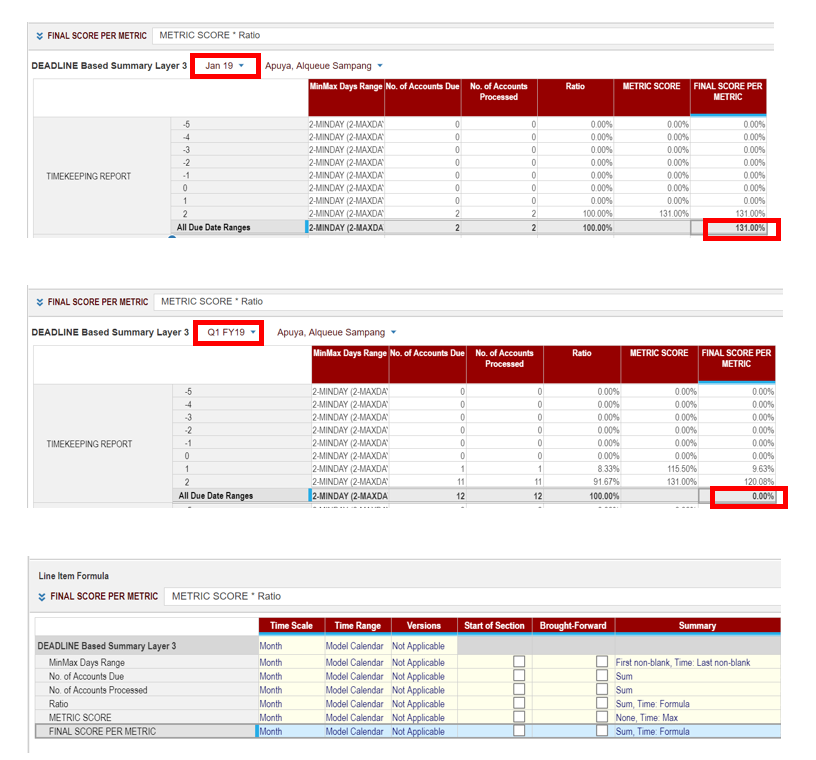Tagged:

•Best solution I could think of is, but not very beautiful either: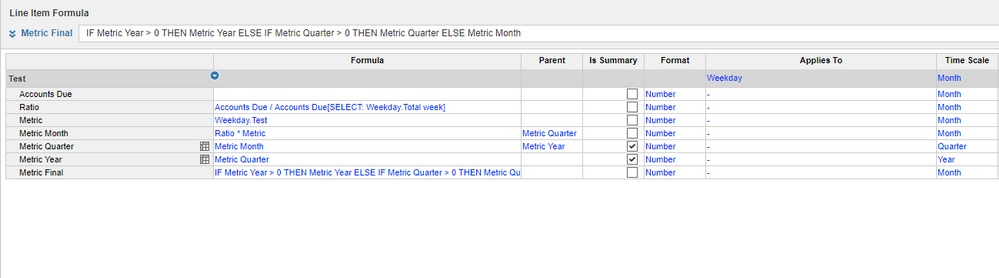The idea is that you hide Metric Month, Metric Quarter & Metric year from your dashboard views. Metric final should display the figures as you want them to be for Months, quarters & years.

•HI @SarahE ,

Please make the summary of Time to 'Sum' or 'Same as Main summary'. In the screen shot I can see the Time summary as "Formula" hence the result is based on Top level item's data present in your source module.

I hope this helps.

Regards,

kavin.

•Hi Sara,

Change the summary of Metric Score to Sum,Time:Max.

~Vignesh M

•@VIGNESH.M here is the result after changing Metric Score to Metric Score to Sum,Time:Max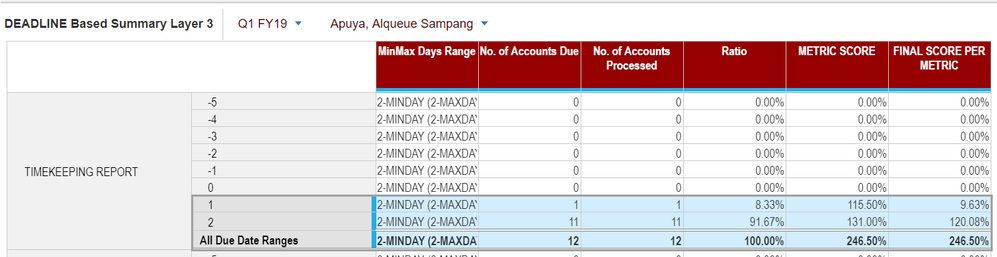•It still doesn't provide the needed result. I am thinking that Summary in Anaplan has its limitation/very limiting so I might result to making another module with Quarter only time dimension but I am not sure if its the best practice to do,

Here is the result after editing the summary: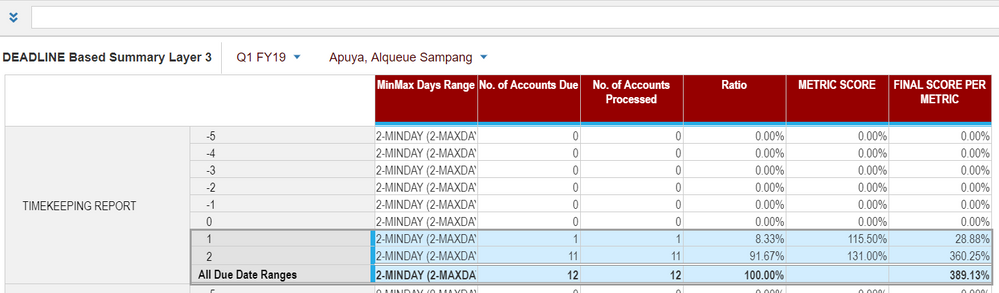•Hi Sara,

We are seeing the numbers, but am not sure about the answer,

Why are you keeping Final Score metric line item Time summary as Formula?

Why don't we change it to Time summary as SUM?

~Vignesh M

•Here is the idea of the requirement:

MinMax Days Range =  Metric Score table applicable (via lookup)

No. of accounts Due = Number of accounts needs to be processed

No. of accounts processed = Number of accounts actually processed

Ratio = Number of Processed over Number of due ratio

Metric Score = Corresponding Metric Score per day range in the MinMax Days metric table (via lookup)

Final Score per Metric = Metric Score multiplied by ratio (to get the weighted score depending on the ratio and metric score)

Final Score per Metric SUM per month is okay since its their score for the month, but per Quarter/Time Summary, the score should be still at Metric Score * Ratio for the quarter hence the FORMULA in Time Summary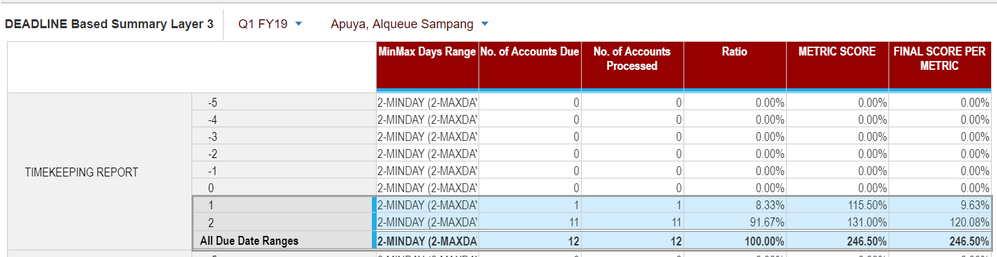•Meantime, what I did is, I created a Time range without Quarter totals and use it to calculate every month data then created another module to calculate quarter data. But I keep on trying to do a single module with Quarterly totals because I think that my current idea is not the best practice.
•Can you please make the Metric Score line item summary as Max,Time: Max. I hope this should resolve your issue. Let me know if it doesn't work.

Regards,

Kavin.

•EDIT:

Sorry I finally understand your request.

- Time should be a formula summary

- Items should be a sum summary

But a sum on items on a higher level of Time should in your case not be a formula, but a sum over the formula items, while Anaplan is doing a formula.

Ill come back with a better solution if there is any.

•I will try to do this setting and I hope it will work, I will get back to all of you as soon as I get the result I need. Thank you!

•It worked!!! This is the result that I need! So now I can delete my QTR modules, and use this instead. It may not be beautiful to look at, as you mentioned, but this is the result I need so thank you 🙂 If I thought of any enhancement on this, I will let you know right away 🙂 thank you! this helped a lot 🙂